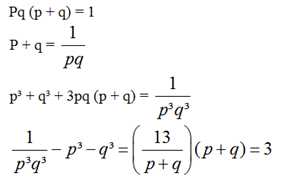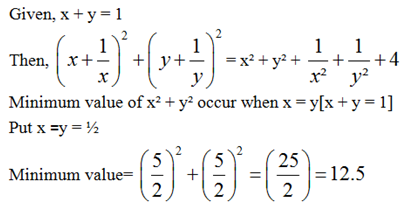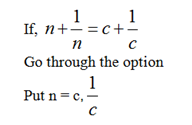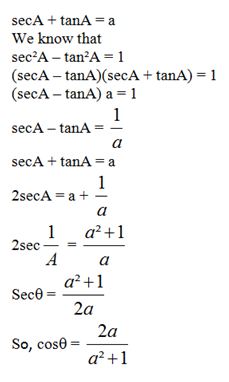# SSC Quantitative Aptitude Questions – Practice Day 1 Questions

Dear Aspirants, Here we have given the Important SSC Exams 2020 Practice Test Papers. Candidates, those who are preparing for SSC CGL, SSC MTS, SSC CHSL Exams 2020 can practice these questions to get more confidence to Crack SSC CGL Exams 2020 Examination.

#### SSC Quantitative Aptitude Day 1 Questions

1) The average weight of A, B and C is x kg. A and C lost y kg each after dieting and B gains kg. After this their average weight decreases by 1 kg. Find y.

a) 1 Kg

b) 2 kg

c) 3 kg

d) Cannot be determined

2) A vessel is full of refined oil. ¼ of the refined oil is taken out and the vessel is filled with mustard oil. If the process is repeated 4 times and 10 liters of refined oil is finally left in the vessel, what is the capacity of the vessel?

a) 33 liters

b) 2460/ 81 liters

c) 2560/81 liters

d) 30 liters

3) In an election of 3 candidates A, B and C, A gets 50% more votes than B. A also beats C by 18,000 votes. If it is known that B gets 5 percentage more votes than C, find the number of voters on the voting list (given 90%) of the voters on the voting list voted and no votes were illegal)

a) 72,000

b) 81,000

c) 90,000

d) 1, 00,000

4) A trader marked his goods at 20% above the cost price. He sold half of the stock at the marked price, one quarter at a discount of 20% on the marked price and the rest at a discount of 40% on the marked price. His total gain is

a) 2%

b) 4.5%

c) 13.5%

d) 15%

5) A trader wants 10% profit on the selling price of a product whereas his expenses amount to 15% on sales. What should be his rate of mark up on an article costing Rs.9?

a) 20%

b) 66 2/3%

c) 30%

d) 100/3%

6) A sum was put at simple interest at a certain rate for 2 years. Had it been put at 1% higher rate, it would have fetched Rs 24 more. What is the sum?

a) 1200

b) 1500

c) 1800

d) 2000

7) Rs 1104 is divided between 3 men, 4 women and 6 boys, so that the share of a man, a woman and a boy are in the proportion of 3 : 2 : 1. How much does each boy get?

a) Rs. 48

b) Rs. 64

c) Rs. 96

d) Cannot be determine

8) ‘X’ number of men can finish a piece of work in 30 days. If there were 6 men more, the work could be finished in 10 days less. The original number of men is:

a) 24 men

b) 12 men

c) 36 men

d) 48 men

9) Wheels of diameter 7 cm and 14 cm start rolling simultaneously from X and Y which are 1980 cm apart towards each other in opposite directions. Both of them make the same number of revolutions per second. If both of them meet after 10 seconds, the speed of the smaller wheel is:

a) 22cm/s

b) 44 cm/s

c) 66 cm/s

d) 132 cm/s

10) A sum of Rs 8000 borrowed at 5% p.a. compound interest and paid back in 3 equal annual installments. What is the amount of each installment?

a) Rs 2937.67

b) Rs 3000

c) Rs 2037.67

d) Rs 2739.76

11) Seats of Physics, Chemistry and Mathematics in a school are in the ratio 4 : 5 : 6. There is a proposal to increase these seats by 75 in each department. What are the total numbers of seats in the school finally?

a) 600

b) 750

c) 900

d) Cannot be determine

12) If pq (p + q) = 1, then the value of 1/ P3q3 – p3 – q3 is equal to

a) 1

b) 2

c) 3

d) 4

13) Let x, y be two positive numbers such that x + y = 1. Then the minimum value of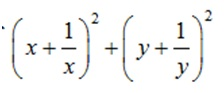a) 12

b) 12.5

c) 20

d) 13.3

14) If x + 1/x = c + 1/c, then the value of x

a) c, 1/c

b) c, c2

c) c, 2c

d) 0, 1

15) If SecA + tanA = a, cosA= ?

a) (a2 + 1)/2a

b) 2a / (a2 + 1)

c) (a2 – 1)/2a

d) 2a/(a2 – 1)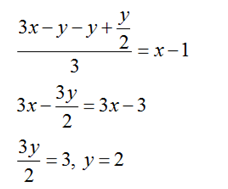Let the quantity of refined oil initially be Q.

Then we have Q × ¾ × ¾ × ¾ × ¾ = 10

Q× ¾ × ¾ × ¾ × ¾ = 10

So, Q = 2560/81 liters

The only values that fit this situation are C 25%, B 30%, and A 45%.

These are the percentage of votes polled. (Note: these values can be got either through trial and error or through solving c + c + 5 + 1.5(c + 5) = 100%

Then, 20%is 18000 (the difference between A & C.)

Hence, 90000 people must have voted and 100000 people must have been on the voter’s list.

Let C.P. of whole stock = Rs. 100,

Then, marked price of whole stock = Rs.120.

M.P. of 1/2 stock = Rs 60, M.P. of 1/4 stock = Rs 30/

So, Total S.P. = Rs [60 + (80% of 30) + (60% of 30)]

Rs (60 + 24 + 18) = Rs 102

Hence, gain% = (102 – 100%) = 2%

= (102 – 100) % = 2%

Let the SP of the article be Rs. x

Expenses= 15% of x =            Rs 0.15x

Profit = 10% of x = Rs 0.10x

CP = Rs 9 (given)

Therefore, 9 + 0.15x + 0.1x   x   12

So, % increase for marked price

= (12 – 9)/ 9 * 100 = 100/ 3 %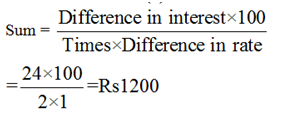Let each boy gets x, so the women gets 2x and a man gets 3x.

Now, (3 × 3x) + (4 × 2x) + (6 × x) = 1104

= 23x = 1104, = x = 48

So, each boy gets Rs 48

X men × 30 days = (x + 6 men) × (30 – 10) days

3X = 2X + 12

X = 12 men

Circumference of the wheel starting from Y

Let both the wheels make n revolution in one second.

Distance covered by both the wheels in 1 sec

= 22n + 44n = 66 n cm

= Distance covered by both the wheels in 10 sec

= 660n cm

Now, 660n = 1980, So, n = 3

Speed of the smaller wheel

= 22 n/cm/s = 66 cm/s

Let the repayment annually be X

Then:

8000 + 3 years interest on 8000

(On compound interest of 5%)

= X + 2 years interest on X + X +

1 year interest on X + X => X = 2937.67

Let the number of seats in Physics, Chemistry and Mathematics be 4x, 5x and 6x.

New ratio of seats

= (4x + 75) : (5x + 75) : (6x + 75)

So, the given data is insufficient.